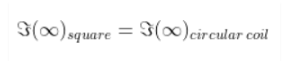# Consider a circular current-carrying

Question:

Consider a circular current-carrying loop of radius R in the x-y plane with centre at the origin. Consider the line integral

$\Im(L)=\left|\int_{-L}^{L} B \cdot d l\right|$ taken along z-axis.

(a) show that

$\Im(L)$ monotonically increases with $\mathrm{L}$

(b) use an appropriate Amperian loop to that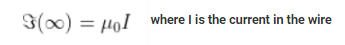(c) verify directly the above result

(d) suppose we replace the circular coil by a square coil of sides R carrying the same current I. What can you say about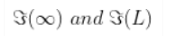Solution: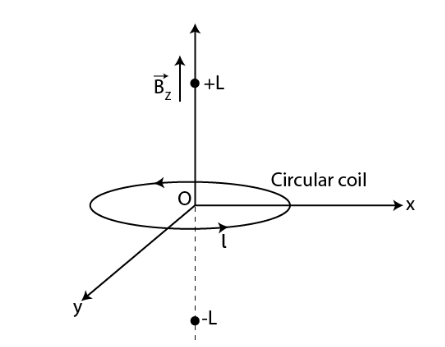(a) Magnetic field due to a circular current-carrying loop is given as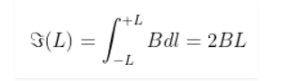It is a monotonically increasing function of L

(b) The Amperian loop is given as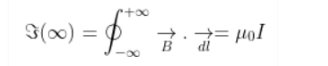(c) The magnetic field at the axis of the circular coil is given as μ0I

(d) When a circular coil is replaced with a square coil, it is given as